# Web技术实现移动监测的介绍

更新时间：2017年09月18日 16:25:27   作者：凹凸实验室我要评论

Web技术实现移动监测的介绍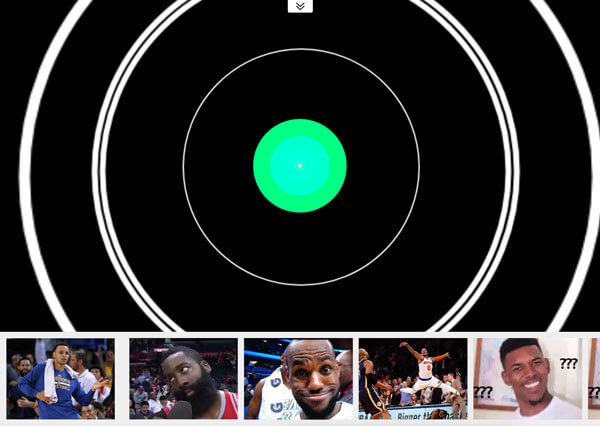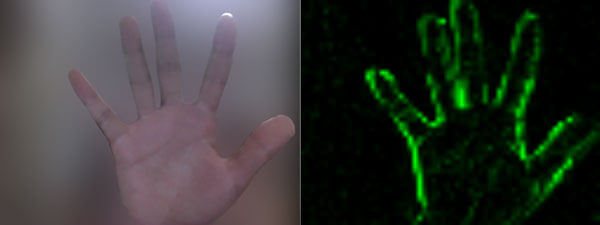```<!-- 若不加 autoplay，则会停留在第一帧 -->
<video id="video" autoplay></video>
// 具体参数含义可看相关文档。
const constraints = {
audio: false,
video: {
width: 640,
height: 480
}
}
.then(stream => {
// 将视频源展示在 video 中
video.srcObject = stream
})
.catch(err => {
console.log(err)
})```

```const video = document.getElementById('video')
const canvas = document.createElement('canvas')
const ctx = canvas.getContext('2d')
canvas.width = 640
canvas.height = 480
// 获取视频中的一帧
function capture () {
ctx.drawImage(video, 0, 0, canvas.width, canvas.height)
// ...其它操作
}```

```function diffTwoImage () {
// 设置新增元素的合成方式
ctx.globalCompositeOperation = 'difference'

// 清除画布
ctx.clearRect(0, 0, canvas.width, canvas.height)

// 假设两张图像尺寸相等
ctx.drawImage(firstImg, 0, 0)
ctx.drawImage(secondImg, 0, 0)
}```1. 当你不知道设计师前后两次给你的设计稿有何差异时
2. 想查看两个浏览器对同一个网页的渲染有何差异时何时为一个“动作”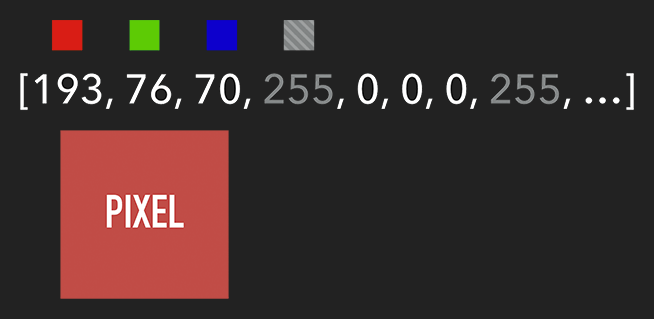```

let imageScore = 0
const rgba = imageData.data
for (let i = 0; i < rgba.length; i += 4) {
const r = rgba[i] / 3
const g = rgba[i + 1] / 3
const b = rgba[i + 2] / 3

const pixelScore = r + g + b

// 如果该像素足够明亮
if (pixelScore >= PIXEL_SCORE_THRESHOLD) {
imageScore++
}
}
// 如果明亮的像素数量满足一定条件
if (imageScore >= IMAGE_SCORE_THRESHOLD) {
// 产生了移动
}```

```
const rgba = imageData.data
for (let i = 0; i < rgba.length; i += 4) {
rgba[i] = 0 // red
rgba[i + 2] = 0 // blue
}
ctx.putImageData(imageData, 0, 0)```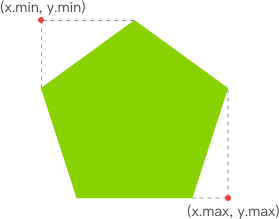```function processDiff (imageData) {
const rgba = imageData.data

let score = 0
let pixelScore = 0
let motionBox = 0

// 遍历整个 canvas 的像素，以找出明亮的点
for (let i = 0; i < rgba.length; i += 4) {
pixelScore = (rgba[i] + rgba[i+1] + rgba[i+2]) / 3

// 若该像素足够明亮
if (pixelScore >= 80) {
score++

coord = calcCoord(i)
motionBox = calcMotionBox(montionBox, coord.x, coord.y)
}
}

return {
score,
motionBox
}
}
// 得到左上角和右下角两个坐标值
function calcMotionBox (curMotionBox, x, y) {
const motionBox = curMotionBox || {
x: { min: coord.x, max: x },
y: { min: coord.y, max: y }
}
motionBox.x.min = Math.min(motionBox.x.min, x)
motionBox.x.max = Math.max(motionBox.x.max, x)
motionBox.y.min = Math.min(motionBox.y.min, y)
motionBox.y.max = Math.max(motionBox.y.max, y)
return motionBox
}
// imageData.data 是一个含有每个像素点 rgba 信息的一维数组。
// 该函数是将上述一维数组的任意下标转为 (x,y) 二维坐标。
function calcCoord(i) {
return {
x: (i / 4) % diffWidth,
y: Math.floor((i / 4) / diffWidth)
}
}```

```ctx.lineWidth = 6
ctx.strokeRect(
diff.motionBox.x.min + 0.5,
diff.motionBox.y.min + 0.5,
diff.motionBox.x.max - diff.motionBox.x.min,
diff.motionBox.y.max - diff.motionBox.y.min
)```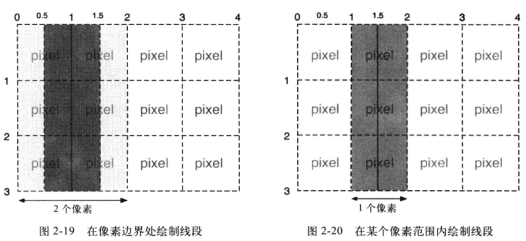```const motionCanvas // 展示给用户看
const backgroundCanvas // offscreen canvas 背后处理数据
motionCanvas.width = 640
motionCanvas.height = 480
backgroundCanvas.width = 64
backgroundCanvas.height = 48```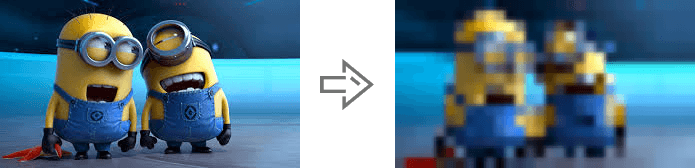• DepthJS：以浏览器插件形式提供数据访问。
• Node-Kinect2： 以 Nodejs 搭建服务器端，提供数据比较完整，实例较多。
• ZigFu：支持 H5、U3D、Flash，API较为完整。
• Kinect-HTML5：Kinect-HTML5 用 C# 搭建服务端，提供色彩数据、深度数据和骨骼数据。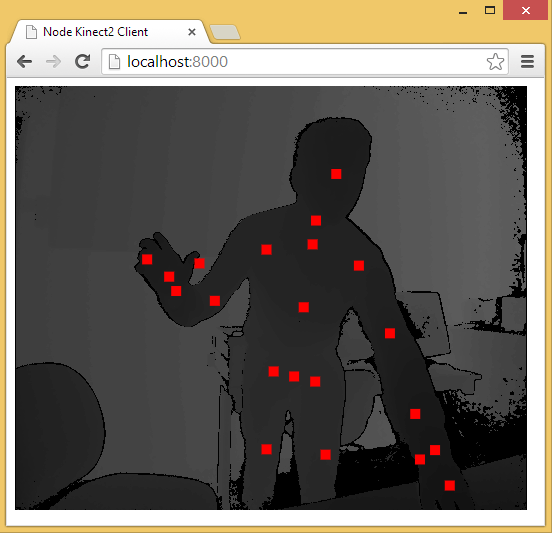MOTION DETECTION WITH JAVASCRIPT